# Sequent calculus

(diff) ← Older revision | Latest revision (diff) | Newer revision → (diff)

One of the formulations of the predicate calculus. Due to the convenient representation of derivations, the sequent calculus has wide applications in proof theory, in the foundations of mathematics and in the automatic search for a deduction. The sequent calculus was introduced by G. Gentzen in 1934 (see ). One version of the classical calculus of predicates in the form of the sequent calculus is presented below.

A collection of formulas is a finite setof formulas of a certain logico-mathematical language, where in this set, repetitions of formulas are permitted. The order of the formulas inis inessential, but for each formula, the number of copies of it that are inis given. The collection of formulas can be empty. The set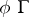is obtained fromby adjoining a copy of the formula. For two collections of formulasand, a figure of the formis called a sequent;is called the antecedent andthe successor of the sequent.

The axioms of the sequent calculus have the form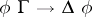, whereandare arbitrary collections of formulas andis an arbitrary atomic (elementary) formula. The derivation rules of the calculus have a very symmetrical structure; logical connectives are introduced into the sequent calculus by the following schemata: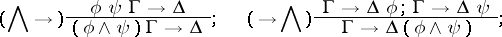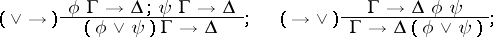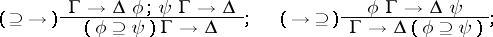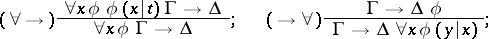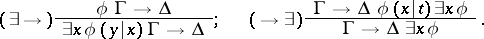In the rulesandit is assumed that the variableis not free inor, and thatis not free in.

The sequent calculus is equivalent to the usual form of predicate calculus, in the sense that a formulais deducible in the predicate calculus if and only if the sequent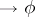is deducible in the sequent calculus. The fundamental theorem of Gentzen (or the normalization theorem) is essential for the proof of this assertion; this theorem can be stated as follows: If the sequents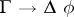and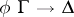are deducible in the sequent calculus, then so is. The derivation rule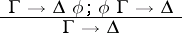is called the cut rule, and the normalization theorem asserts that the cut rule is admissible in the sequent calculus, or that the addition of the cut rule does not change the collection of deducible sequents. In view of this, Gentzen's theorem is also called the cut-elimination theorem.

The symmetric structure of the sequent calculus greatly facilitates the study of its properties. Therefore, an important place in proof theory is occupied by the search for sequent variants of applied calculi: arithmetic, analysis, type theory, and also the proof for such calculi of the cut-elimination theorem in one form or another (see , ). Sequent variants can also be found for many calculi based on non-classical logics: intuitionistic, modal, relevance logics, and others (see , ).